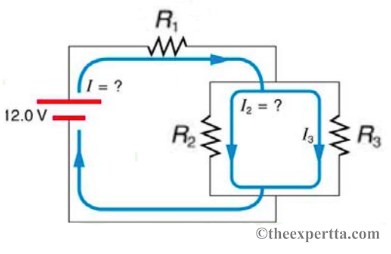# Problem: In the figure, these three resistors are connected to a voltage source so that R2 = 5.75 Ω and R3 = 11.5 Ω are in parallel with one another and that combination is in series with R1 = 1.75 Ω. (a) Calculate the power being dissipated by the third resistor P3, in watts.(b) Find the total power supplied by the source, in watts.

###### FREE Expert Solution###### Problem Details

In the figure, these three resistors are connected to a voltage source so that R2 = 5.75 Ω and R3 = 11.5 Ω are in parallel with one another and that combination is in series with R1 = 1.75 Ω.(a) Calculate the power being dissipated by the third resistor P3, in watts.

(b) Find the total power supplied by the source, in watts.